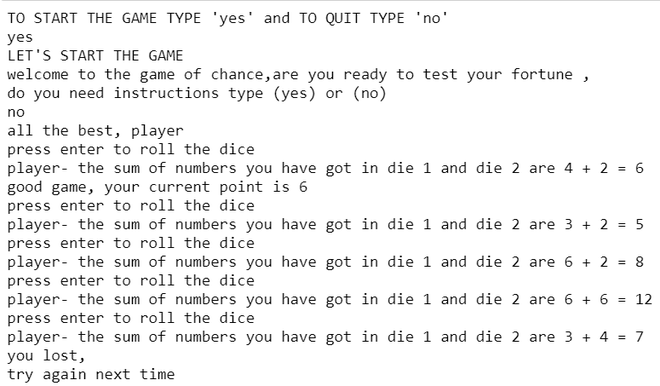Skip to content
Related Articles
Game of Craps in Python
• Difficulty Level : Easy
• Last Updated : 24 Jan, 2021

In this article, we are going to discuss how to create the Game of Craps using Python.

Rules of the game:

1. Two dices are required to play and a player rolls two six-sided dice and adds the numbers rolled together.
2. If on the first roll a player encounters a total of 7 or 11 the player automatically wins, and if the player rolls a total of 2, 3, or 12 the player automatically loses, and play is over.
3. If a player rolls a total of 4, 5, 6, 8, 9, or 10 on their first roll, that number becomes the ‘point. Then the player continues to roll the two dice again until one of two things happens either they roll the ‘point’ again, in which case they win, or they roll a 7, in which case they lose.

Approach:

• When you run the program at first you get a choice on whether to start the game or exit from the game and this is done by importing the sys module after you continue to play the game, you will get an option to view the rules of the game or if you are already familiar with the rules you can choose not to see them.
• When you start the game by pressing enter the random module choose two numbers between 1 and 6 randomly. Then by the use of diceNumber() function, the two numbers are added together.
• Now by following the rules of the game if the total of your dices are 7 or 11 you win. And if you score a total of 2,3,12 you will automatically lose.
• And if your total is 4,5,6,8,9 or 10 that total will be saved and the program will run in a loop until two things happen 1)you score the same numbers as before or you score 7. If you have scored the same number as before you will win and if you have scored a total f 7 you have lost.

Implementation:

## Python3

 `# import required modules``import` `random      ``import` `sys`` ` `# stat the game``a ``=` `input``(``"TO START THE GAME TYPE 'yes' and TO QUIT TYPE 'no'\n"``)``if` `a.lower() ``=``=` `"no"``:``    ``sys.exit()``else``:``    ``print``(``"LET'S START THE GAME"``)`` ` `     ` `# those who need instructions can ask for it, ``# others can start the game directly.``a ``=` `input``(``"welcome to the game of chance,are you ready to test your fortune ,\ndo you need instructions type (yes) or (no) \n"``)`` ` `if` `a.lower() ``=``=` `"yes"``:``    ``print``(``''' 1. player rolls two six-sided dice and adds the numbers rolled together.``              ``2. On this first roll, a 7 or an 11 automatically wins, and a 2, 3, or 12automatically loses, and play is over.``                 ``If a 4, 5, 6, 8, 9, or 10 are rolled on this first roll, that number becomes the 'point.'``              ``3. The player continues to roll the two dice again until one of two things happens: ``                 ``either they roll the 'point' again, in which case they win; or they roll a 7, in which case they lose.'''``)`` ` `elif` `a.lower() ``=``=` `"no"``:``    ``print``(``"all the best, player"``)`` ` ` ` `# function to generate dice throws    ``def` `diceNumber():``   ` `    ``_ ``=` `input``(``"press enter to roll the dice "``)``     ` `    ``# this will enable to select a ``    ``# random number from 1 to 6``    ``die1 ``=` `random.randrange(``1``, ``7``)``    ``die2 ``=` `random.randrange(``1``, ``7``)``     ` `    ``# returns the diceNumber values ``    ``# in the form of tuple``    ``return` `(die1, die2)  `` ` `# function to get dice sum  ``def` `twoDice(dices):``    ``die1, die2 ``=` `dices``    ``print``(``"player- the sum of numbers you have got in die 1 and die 2 are {} + {} = {}"``.``format``(die1, die2, ``sum``(dices)))`` ` ` ` `# calling the diceNumber function to get a value``# return the roll and then store that ``# value in value.``value ``=` `diceNumber()``twoDice(value)`` ` `# using the sum function in value to ``# find the sum of two outcomes.``sum_of_dices ``=` `sum``(value)`` ` ` ` `# find if sum of dices is 7 or 11 to determine the result.``if` `sum_of_dices ``in` `(``7``, ``11``):``    ``result ``=` `"congratulations you won"`` ` `# find if sum of dices is 2 , 3 , 12 to determine the result.``elif` `sum_of_dices ``in` `(``2``, ``3``, ``12``):``    ``result ``=` `"you lost, \ntry again next time"``     ` `# if none of the cases worked above now we will ``# play continously until we win or lose.    ``else``:  ``    ``result ``=` `"continue your game please"``    ``currentpoint ``=` `sum_of_dices``    ``print``(``"good game, your current point is"``, currentpoint)`` ` ` ` `# game continues if you have not scored a ``# total of 2 , 3 , 7 , 11 , 12 this will ``# enable the game to continue in a loop until``# the outcome is win or lose``while` `result ``=``=` `"continue your game please"``:``    ``value ``=` `diceNumber()``    ``twoDice(value)``    ``sum_of_dices ``=` `sum``(value)``     ` `    ``if` `sum_of_dices ``=``=` `currentpoint:``        ``result ``=` `"congratulations you won"``         ` `    ``elif` `sum_of_dices ``=``=` `7``:``        ``result ``=` `"you lost,\n try again next time"`` ` `# when the outcome is clear,this will produce the ``# outcome of the game``if` `result ``=``=` `"congratulations you won"``:``    ``print``(``"congratulations,you won"``)``     ` `else``:``    ``print``(``"you lost, \ntry again next time"``)`

Output:Attention geek! Strengthen your foundations with the Python Programming Foundation Course and learn the basics.

To begin with, your interview preparations Enhance your Data Structures concepts with the Python DS Course. And to begin with your Machine Learning Journey, join the Machine Learning – Basic Level Course

My Personal Notes arrow_drop_up